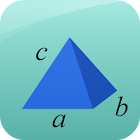Area and Volume Calculator

• 검색 유형

모든 Android 애플리케이션 카테고리

모든 Android 게임 카테고리# Area and Volume Calculator

by: 2.2K 7.8

7.8 사용자
평가 등급

## 스크린샷

설명

This free app is a geometric calculator which is able to calculate area and volume.

Area Calculator: You are able to calculate the area for the most important geometric figures. You can calculate the area of circle, ellipse, rectangle, square, trapezoid, triangle, parallelogram, rhombus, sector and triangle.

Volume Calculator: You are able to calculate the volume for the most important geometric objects. You can calculate the volume of cone, cube, cuboid, cylinder, sphere, square pyramid and tetrahedron.

Best math tool for school and college! If you are a student, it will help you to learn geometry!

: 2.158 리뷰

"우수"

7.8